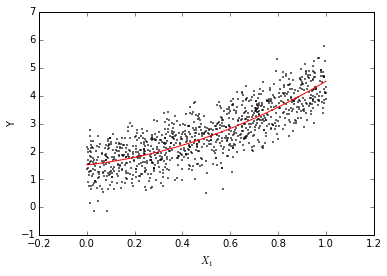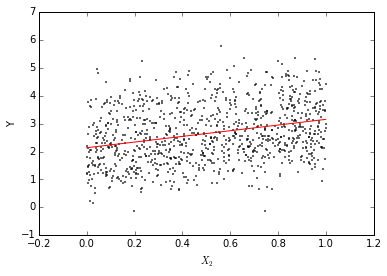Main Effect Plots¶

Main Effect Plots are graphical devices used to visualize the fitted relationship between an independent variable and the dependent one in a regression model. They can be used in any setup, but they're particularly useful when non-linear specifications are used.

In this notebook, we'll walk through an example to visualize a simple model with two explanaory variables, where one of them enters the model with both a linear and a squared term.

In :
%matplotlib inline

import matplotlib.pyplot as plt
import numpy as np
import pandas as pd
import pysal as ps

np.random.seed(123)
• DGP:
In :
xs = ['x1', 'x1a', 'x2']

db = pd.DataFrame(np.random.random((1000, 2)), columns=['x1', 'x2'])
db['x1a'] = db['x1']**2
db['c'] = 1
db['y'] = np.dot(db[['c']+xs].values, np.array([[1., 1., 2., 1.]]).T) \
+ np.random.normal(size=(1000, 1), scale=0.5)
• Model:
$$y = \alpha + \beta_{1a} X_1 + \beta_{1a} X_1^2 + \beta_2 X_2 + \epsilon$$
In :
ols = ps.spreg.OLS(db[['y']].values, db[xs].values, \
name_x=xs, nonspat_diag=False)
print ols.summary
REGRESSION
----------
SUMMARY OF OUTPUT: ORDINARY LEAST SQUARES
-----------------------------------------
Data set            :     unknown
Dependent Variable  :     dep_var                Number of Observations:        1000
Mean dependent var  :      2.6528                Number of Variables   :           4
S.D. dependent var  :      1.0374                Degrees of Freedom    :         996
R-squared           :      0.7745

------------------------------------------------------------------------------------
Variable     Coefficient       Std.Error     t-Statistic     Probability
------------------------------------------------------------------------------------
CONSTANT       1.0049370       0.0526550      19.0853071       0.0000000
x1       0.9428275       0.2136614       4.4127185       0.0000113
x1a       2.0536484       0.2085346       9.8480001       0.0000000
x2       1.0178299       0.0526472      19.3330262       0.0000000
------------------------------------------------------------------------------------
================================ END OF REPORT =====================================
• Plots
In :
rng = np.linspace(db['x1'].min(), db['x1'].max(), 100)
h = (rng * ols.betas) + (rng**2 * ols.betas) + db.x2.mean()*ols.betas + ols.betas
plt.plot(rng, h, c='red')
plt.scatter(db['x1'], db['y'], c='k', s=0.5)
plt.xlabel('$X_1$')
plt.ylabel('Y')
plt.show()In :
rng = np.linspace(db['x2'].min(), db['x2'].max(), 100)
h = (rng * ols.betas) + db.x1.mean()*ols.betas + \
(db.x1**2).mean()*ols.betas + ols.betas
plt.plot(rng, h, c='red')
plt.scatter(db['x2'], db['y'], c='k', s=0.5)
plt.xlabel('$X_2$')
plt.ylabel('Y')
plt.show()